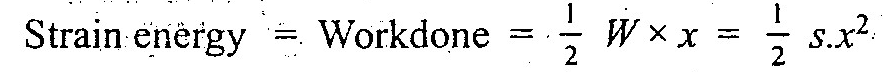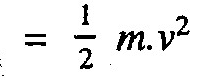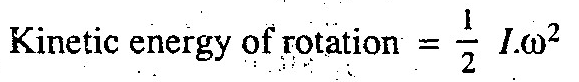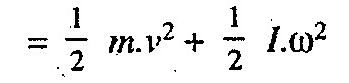### Mechanical Energy | Definition | Types

What is Mechanical Energy? How do we define it and what are different types of it?

It is the capacity of doing work. The mechanical energy is equal to the workdone on a body in altering either its position or its velocity. Following are the three types of mechanical energies:

1. Potential energy: It is the energy possessed by a body for doing work, by virtue of its position.

Let
m = Mass of the body,
W = Weight of the body = m.g, and
h = Distance through which the body falls.
Potential energy = W.h = m.g.h

2. Strain energy: It is the potential energy stored by an elastic body when deformed. A compressed spring possesses this type of energy, because it can do some work in recovering its original shape. Thus if a compressed spring of stiffness S newton per unit extension or compression, is deformed through a distance x by a load W, then

Strain energy = Workdone3. Kinetic energy: It is the energy possessed by a body, for doing work, by virtue of its mass (m) and velocity of motion (v). Mathematically, kinetic energy of the body or kinetic energy of translation,'Notes:

1. When a body of mass moment of inertia (I) about a given axis is rotated about that axis with an angular velocity ω, then it possesses some kinetic energy. In this case,

Kinetic energy of rotation is given by:2. When the body has both linear and angular motions e.g. in the locomotive driving wheels and wheels of a moving car, then total kinetic energy of the body